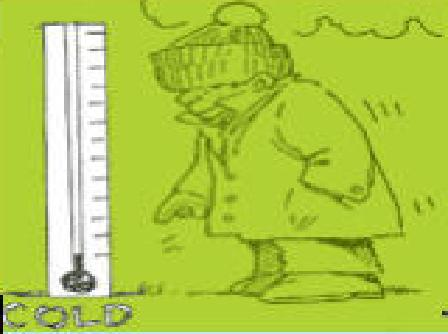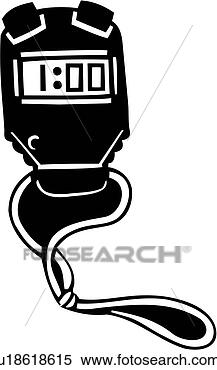MATH!

MATH

Roman Numerals

Convert ANY number to Roman NumeralsNegative Numbers: Ordering NumbersMinute Math: Practice your math and improve your Minute Math score with this game: Quick CalculateCoordinate Planes Games!

Billy Bug Game-Easy

What's the Point?

Using a Coordinate Grid

Stock the Shelves!GEOMETRY

Fun computer activities about all things geometry!

Sum of Interior Angles

Measuring Angles

Do you know your Geometry Terms?

Geometric Nets

Geometric Solids!